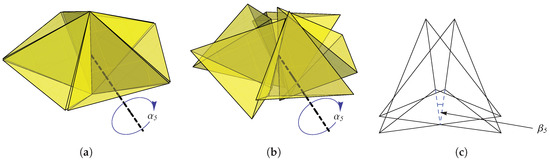Next Article in Journal
The Unexpected Fractal Signatures in Fibonacci Chains
Article

# Cabinet of Curiosities: The Interesting Geometry of the Angle β = arccos ((3ϕ − 1)/4)

Quantum Gravity Research, Topanga, CA 90290, USA
*
Author to whom correspondence should be addressed.
Fractal Fract. 2019, 3(4), 48; https://doi.org/10.3390/fractalfract3040048
Received: 12 September 2019 / Revised: 26 October 2019 / Accepted: 27 October 2019 / Published: 30 October 2019
In this paper, we present the construction of several aggregates of tetrahedra. Each construction is obtained by performing rotations on an initial set of tetrahedra that either (1) contains gaps between adjacent tetrahedra, or (2) exhibits an aperiodic nature. Following this rotation, gaps of the former case are “closed” (in the sense that faces of adjacent tetrahedra are brought into contact to form a “face junction”), while translational and rotational symmetries are obtained in the latter case. In all cases, an angular displacement of $β = arccos 3 ϕ − 1 / 4$ (or a closely related angle), where $ϕ = 1 + 5 / 2$ is the golden ratio, is observed between faces of a junction. Additionally, the overall number of plane classes, defined as the number of distinct facial orientations in the collection of tetrahedra, is reduced following the transformation. Finally, we present several “curiosities” involving the structures discussed here with the goal of inspiring the reader’s interest in constructions of this nature and their attending, interesting properties. View Full-Text
Keywords:
Show FiguresFigure 1

MDPI and ACS Style

Fang, F.; Irwin, K.; Kovacs, J.; Sadler, G. Cabinet of Curiosities: The Interesting Geometry of the Angle β = arccos ((3ϕ − 1)/4). Fractal Fract. 2019, 3, 48. https://doi.org/10.3390/fractalfract3040048

AMA Style

Fang F, Irwin K, Kovacs J, Sadler G. Cabinet of Curiosities: The Interesting Geometry of the Angle β = arccos ((3ϕ − 1)/4). Fractal and Fractional. 2019; 3(4):48. https://doi.org/10.3390/fractalfract3040048

Chicago/Turabian Style

Fang, Fang, Klee Irwin, Julio Kovacs, and Garrett Sadler. 2019. "Cabinet of Curiosities: The Interesting Geometry of the Angle β = arccos ((3ϕ − 1)/4)" Fractal and Fractional 3, no. 4: 48. https://doi.org/10.3390/fractalfract3040048

Find Other Styles
Note that from the first issue of 2016, MDPI journals use article numbers instead of page numbers. See further details here.

1